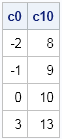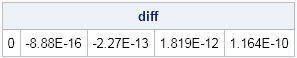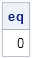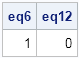One of my favorite new features of SAS/IML 12.1 enables you to define functions that contain default values for parameters. This is extremely useful when you want to write a function that has optional arguments.

### Example: Centering a data vector

It is simple to specify a SAS/IML module with a default parameter value. Suppose that you want to write a module that centers a vector. By default, the module should center the vector so that new mean of the data is 0. However, the module should also support an arbitrary value to center the vector. With default arguments in SAS/IML 12.1, you can write the following module:

```proc iml; start Center(x, a=0); return ( x - mean(x) + a ); finish;```

The Center module has two arguments, but the equal sign in the second argument indicates that the second argument is optional and that it receives a default value of 0. (SAS programmers who are familiar with the macro language will find this syntax familiar.) Using default values enables you to call the function with fewer parameters in the usual (default) case, but also enables you to call the function with more generality.

In this example, if you call the function by using one argument, the local variable a is set to zero. You can also specify a value for a when you call the function, as follows:

```x = {-1,0,1,4}; /* data */ c0 = Center(x); /* center x at 0 */ c10 = Center(x, 10); /* center x at 10 */ print c0 c10;```The c0 vector is centered at 0, whereas the c10 vector is centered at 10. The Center module has a simpler calling syntax for the usual case of centering a vector at 0.

### Example: Testing whether two vectors are equal to within a certain precision

I have previously blogged about the dangers of testing floating-point values for equality. In a numerical program, you should avoid testing whether a vector x is (exactly) equal to another vector y. Even though two vectors should be equal (if the computations were performed in exact arithmetic), finite-precision computations can lead to small differences, which some people call rounding errors. For example, in exact arithmetic the square of the square root of x is exactly equal to x for any nonnegative value. However, the following statements show that this equality might not hold in finite precision arithmetic:

```x = {4 5 2000 12345 654321}; /* some numbers */ y = sqrt(x)#sqrt(x); /* in exact arithmetic, y=x */ diff = x-y; /* the diff vector is not zero */ print diff;```As you can see, some elements of x and y are not equal, which leads to a nonzero difference vector. Consequently, it is a bad idea to use the equal operator (=) to test whether the vectors are equal:

```eq = all(x=y); /* Bad idea: Don't test for exact comparison */ print eq;```To understand more about floating-point arithmetic, see the paper "What Every Computer Scientist Should Know About Floating-Point Arithmetic." A better way to compare numerical values in SAS/IML is to test whether two numbers are within some specified tolerance of each other, like you can do with PROC COMPARE. The following module computes whether the absolute value of the difference between corresponding elements is less than some criterion. By default, the criterion is set to 10–6:

```/* return 1 if x[i] is within eps of y[i] for all i */ start IsEqual(x, y, eps=1e-6); return( all( abs(x-y)<=eps) ); finish;   eq6 = IsEqual(x, y); /* Default: compare within 1e-6 */ eq12 = IsEqual(x, y, 1e-12); /* compare within 1e-12 */ print eq6 eq12;```The vectors are judged to be equal when the default criterion is used. If you tighten the criterion, the vectors are judged to be unequal.

Being able to specify default parameter values is very useful to a programmer. Do you have an example for which this new feature of SAS/IML 12.1 will be useful? Describe your application in the comments.

ShareDistinguished Researcher in Computational Statistics

Rick Wicklin, PhD, is a distinguished researcher in computational statistics at SAS and is a principal developer of SAS/IML software. His areas of expertise include computational statistics, simulation, statistical graphics, and modern methods in statistical data analysis. Rick is author of the books Statistical Programming with SAS/IML Software and Simulating Data with SAS.

1.•